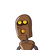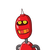# the marks obtained by 10 students of a class are given below 23,18,27,28,18,26,20 find the mean of the marks obtained above ?​

the marks obtained by 10 students of a class are given below 23,18,27,28,18,26,20 find the mean of the marks obtained above ?​

### 2 thoughts on “the marks obtained by 10 students of a class are given below 23,18,27,28,18,26,20 find the mean of the marks obtained above ?​”

1.The Mean = 22.86.

Step-by-step explanation:

Mean = A1+A2+A3+. . . . . An / n

Mean = 23+18+27+28+18+26+20 / 7

Mean = 160/7

Mean = 22.86

2.